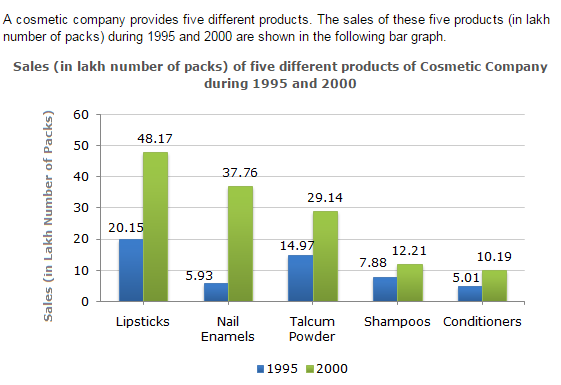Direction1.

The sales of lipsticks in 2000 was by what percent more than the sales of nail enamels in 2000? (rounded off to nearest integer)

 A. 33% B. 31% C. 28% D. 22%
Explanation: Required percentage = [ (48.17 - 37.76) x 100 ] % 37.76 = 27.57% ~= 28%.

2.

During the period 1995-2000, the minimum rate of increase in sales is in the case of?

 A. Shampoos B. Nail enamels C. Talcum powders D. Lipsticks
Explanation: The percentage increase from 1995 to 2000 for various products are: Lipsticks = [ (48.17 - 20.15) x 100 ] % = 139.06%. 20.15 Nail enamels = [ (37.76 - 5.93) x 100 ] % = 536.76%. 5.93 Talcum powders = [ (29.14 - 14.97) x 100 ] % = 94.66%. 14.97 Shampoos = [ (12.21 - 7.88) x 100 ] % = 54.95% ~= 55%. 7.88 Conditioners = [ (10.19 - 5.01) x 100 ] % = 103.39%. 5.01 Therefore The minimum rate of increase in sales from 1995 to 2000 is in the case of Shampoos.

3.

What is the approximate ratio of the sales of nail enamels in 2000 to the sales of Talcum powders in 1995?

 A. 7:2 B. 5:2 C. 4:3 D. 2:1
Explanation: Required ratio = 37.76 ~= 2.5 = 5 . 14.97 2

4.

The sales have increase by nearly 55% from 1995 to 2000 in the case of?

 A. Lipsticks B. Nail enamels C. Talcum powders D. Shampoos